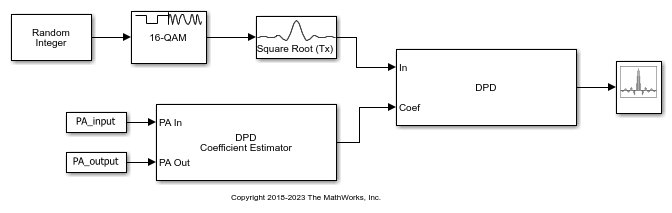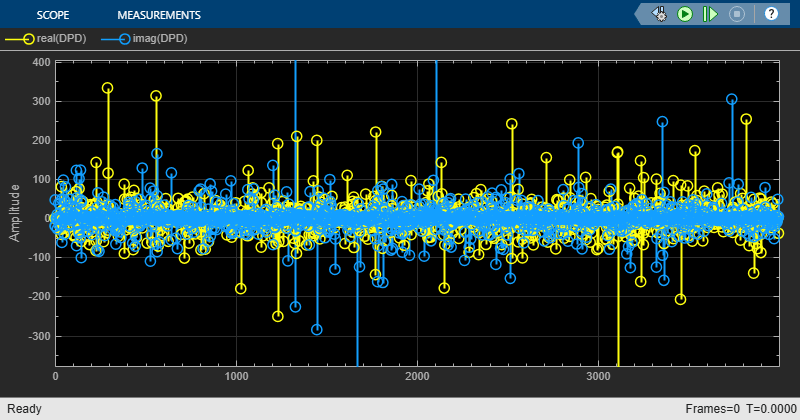# Predistort Power Amplifier Input Signal in Simulink

Apply digital predistortion (DPD) to a 16-QAM signal of random symbols. The DPD Coefficient Estimator block uses a captured signal containing from input and output signals from a power amplifier (PA) to determine the predistortion coefficient matrix. Digital predistortion of the signal preconditions is to correct impairments that the PA introduces. This model does not include a block representing the PA.

The `PreLoadFcn` callback (found in `File>Model Properties>Callbacks`) initializes model parameters, and also loads workspace variables `PA_Input` and `PA_Output` from the file `commpowamp_dpd_data.mat`. The `PA_Input` and `PA_Output` variables are baseband-equivalent signals captured at the input and output of a PA. `PA_Input` and `PA_Output` are used by the DPD Coefficient Estimator block to estimate the memory-polynomial coefficients. The memory-polynomial coefficients are input to the DPD block to predistort the PA input signal.The input signal path of the model generates a random symbol stream, applies 16-QAM modulation, and then applies raised-cosine transmit filtering to the modulated signal.

The input signal is digitally predistorted in the DPD block using the memory-polynomial coefficients generated in the DPD Coefficient Estimator block. The DPD block returns the predistorted input signal for the PA that produced the distorted PA output signal.